## Multiple optimizations passes with case insensitive routing

time to read 5 min | 938 words

The following benchmark is from a small database containing about 500K documents, doing random load by id. As you can see, I highlighted a problematic area: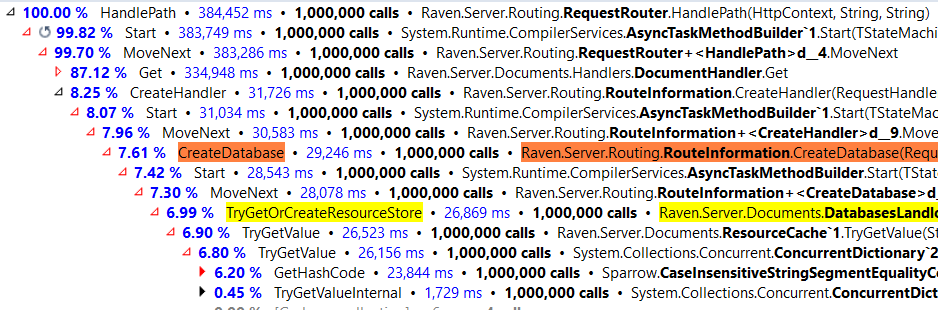We spent a lot of time to optimize routing as much as possible, so I was pretty annoyed when I saw a profiler output showing that we spend 7% – 8% of our time handling routing.

Actually, that is a lie. We spent most of our time looking up what database we should be using.

I decided to simplify the code a lot, to get down to the essentials, and this is the hotspot in question:

We can’t really test this in something like Benchmark.NET, because we need to see how it works when using multiple threads and concurrent access. We care more about the overall performance than a single invocation.

So I tested it by spinning 32 threads that would call the class above (initialized with 10 different values) with the following keys:

• Oren
• oren
• oRen

Each of the threads would process as many of those calls as it can in the span of 15 seconds. And we’ll then tally up the result. The code above gives me 89 million calls per second, which is impressive. Except that this is actually able to utilize the GetCaseInsensitiveHash, which is an internal call (written in C++) that is extremely efficient. On the other hand, my string segment code is far slower.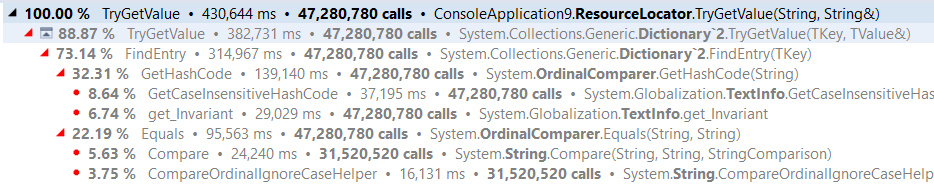On the other hand, if I give up on the OrdinalIgnoreCase, in the code above we get 225 million operations / sec, so there is definitely performance left on the table.

The first attempt was to introduce a bit of smarts, if we have a match by case, we can actually check it and still be faster than the case insensitive version. The code looks like this:

This gave a performance of 76 millions ops / sec when running a mix match, and 205 millions / sec when always using the case matching. That was awesome, but we still missed something. This optimization will kick in only if you actually had an exact case match, but it is very common to miss that. In fact, we noticed that because after we applied this optimization, we created a different benchmark where we got a case mismatch, and had hit the same perf issue.

So the next attempt was to actually learn on the fly. The basic idea is that we still have the two dictionaries, but when we have a miss at the first level, we’ll add the entry to the case sensitive dictionary based on what was searched. In this way, we can learn over time, and then most of the calls would be very fast. Here is the code:

And we get 167 millions ops / second using it.

Moving the code to using a ConcurrentDictionary upped this to 180 millions ops / sec.

And this is the final result of actually implementing this: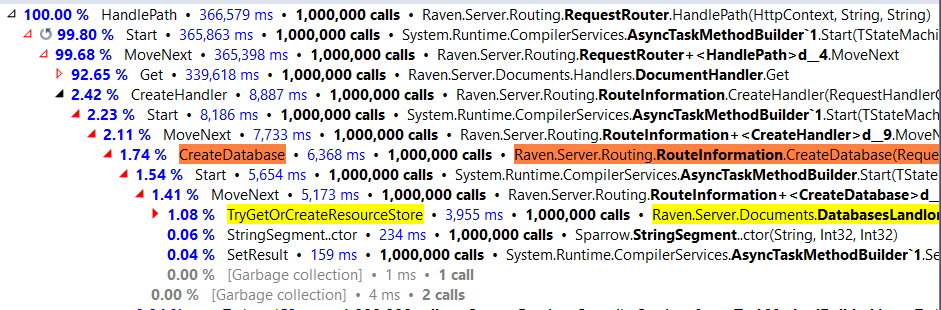Cost went down from 29.2 seconds to 6.3 seconds! There is still significant cost here around using the concurrent dictionary, but drilling down shows that we are stuck: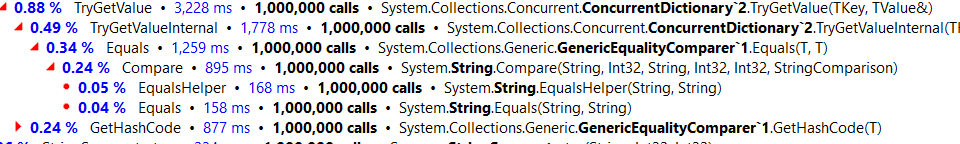This is all high end framework code. But we can do better. Instead of calling the framework, and passing this through multiple chains of calls, we can just compare the memory values directly, like so: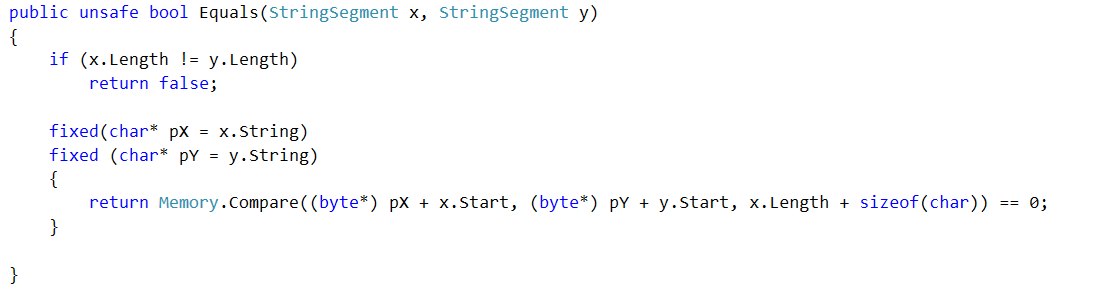And this result in: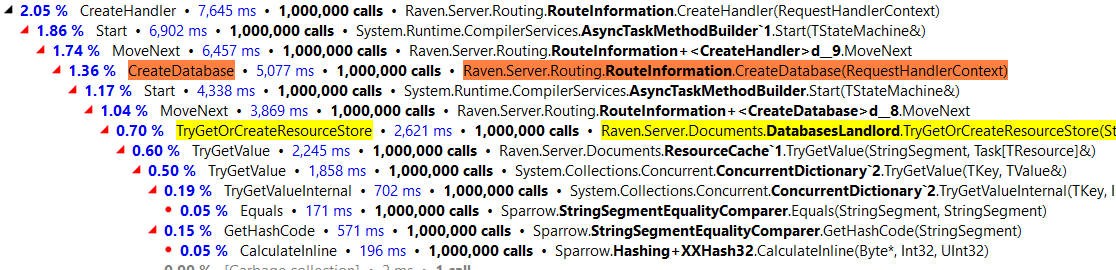So we dropped the cost fro 6.3 (29.2 initially!) seconds to 5 seconds.

Although, let us take a deeper look at this, shall we?It looks like the actual costs we have here for finding the data are now dominated by the call to ResourceCache.TryGetValue. A small change there gave us: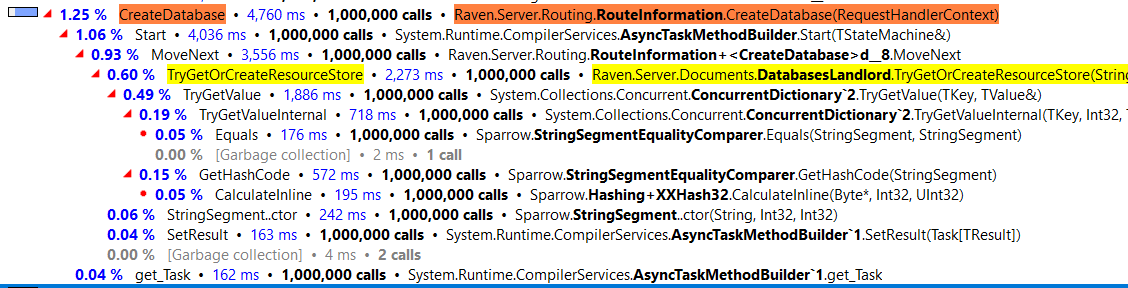So we saved over 250 ms in our test run, and a total of 6.36% of our runtime cost.

What was the change?The parts outlined in yellow are new. So instead of having a pretty big method, we now have a very small one that does the happy case, and the rest is in the unlikely method that will be called rarely.